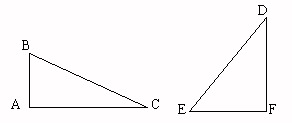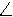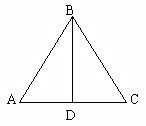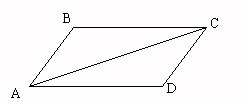Name _____________________________Date ___________________
Geometry
Justify the conclusion by identifying the property as reflexive, transitive, or symmetric.

 1Given:mA = mAmB = mBmC = mCProve: mAmA
 2Given:mA = mFProve: mFmA
 3Given:Line BD bisectsABCmCBDmBADProve:mABDmBAD
 4Given:mA = mAProve: mAmA
 5Given:mA = mCmB = mBProve: mBmB
 6Given:Line AC bisectsBCDmDCAmDACProve:mBCAmDAC